# Archimedes' principle and flotation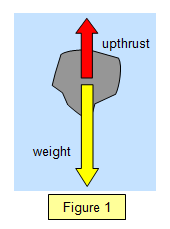You will probably have noticed that objects in water appear to weigh less then when they are in air. For example if you try to lift up a weight in a swimming pool and then try to lift the same weight on the edge of the pool, it feels much lighter in the water.

When an object is in a fluid (such as water) it will displace a certain amount of the fluid. The effect of this is to produce an upward force on the body and the apparent weight of the body is therefore less in the fluid than it would be a vacuum (Figure 1). The magnitude of this upward force, or upthrust, depends on
(a) the volume of the body - the more fluid that is displaced the greater the upthrust;
(b) the density of the fluid - the greater the density the greater the upthrust.

Archimedes, a Greek physicist of the third century BC, is said to have been pondering the problems of flotation while having a bath when he suddenly realised why objects appear to weigh less when immersed in a denser fluid.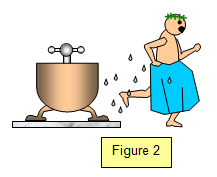The story goes that he leaped from the bath, cried, 'Eureka" (meaning, 'I have it!'), and rushed off naked down the street. More formally, he stated the result in the form that is now known as Archimedes principle:

When a body is wholly or partially immersed in a fluid there is an upthrust which is equal to the weight of fluid displaced.

### Proof of Archimedes principle

Consider a uniform cylinder immersed in a liquid as shown in Figure 3.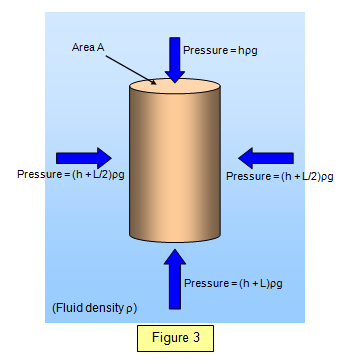Force on the upper face of the cylinder = hρgA
Force on the lower face of the cylinder = [h + L]ρgA
Difference in force = LρgA

But LA is the volume of liquid displaced by the cylinder, and LrgA is the weight of the liquid displaced by the cylinder.

Therefore there is a net upward force on the cylinder equal to the weight of the fluid displaced by it.

The same result will be obtained for a body of any shape, regular or not by taking into account the vertical and horirontal components of the forces on the object.

If a sphere of radius r made of material of density σ is fully immersed in a liquid of density ρ the apparent weight of the sphere is given by:

Apparent weight = actual weight - upthrust = 4/3 πr3g(ρ - s)

The fluid may be a liquid, such as water, or a gas, such as air, although due to the low density of air (about 1 kg m3) the upthrust in air in usually small, but sufficient to support a helium-filled balloon or a hot air balloon.

Example problems
1. A 20 kg spherical hollow steel buoy of volume 0.06 m3 is tethered to the bottom of a fast flowing river by a cable so that the cable makes an angle of 40o with the base of the river. Calculate the tension (T) in this cable.

Resolving vertically and taking g = 9.8 ms-2
Forces on the buoy:
Upthrust = mg+T[cos40] = 20g + T[cos40]
But Upthrust = 0.06 x 1000 x g
Therefore: 20g + T[cos40] = 0.06 x 1000 x g so T cos 40 = 60g – 20g = 40g
T = 511 N

2. A hot air balloon with a volume of 200 m3 hangs in the air. If the density of the hot air is 0.8 kgm-3 and that of the cool air outside the balloon is 1.2 kgm-3 what is the biggest load it can support if the fabric of the balloon and the basket have a total mass of 60 kg. (Take g = 9.8 ms-2)

Weight of balloon and basket = 60 g = 588 N
Upthrust = Weight of air displaced = 200x1.2x g = 2352 N
Weight of hot air in the balloon = 200x0.8x9.8 = 1568 N
Total weight of balloon and hot air = 1568 + 588 = 2156 N
Therefore additional load that can be supported by the balloon = 2352 – 2156 = 196 N

## Floating objects

It should be clear from the above that a floating body will displace its own weight of fluid such that there is no vertical resultant force on the body. The volume of the floating object that is below the surface will depend on both the density of the object and that of the fluid in which it is floating.

Example problems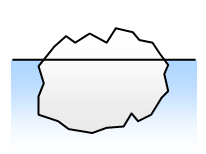Find the volume of an iceberg that is below the surface of sea water.
Density of ice = 920 kgm-3, density of sea water = 1030 kgm-3.
Upthrust on the ice = weight of iceberg = volume of iceberg x density of ice x g = weight of sea water displaced = volume of sea water displaced x density of sea water x g
The volume of sea water displaced is the volume of the iceberg that is beneath the sea.
Therefore: Volume of iceberg x 920 = Volume of iceberg beneath the sea x 1030
So:
Volume of iceberg beneath the sea/Volume of iceberg
= [920/1030] x Volume of iceberg

### Ferries and other ships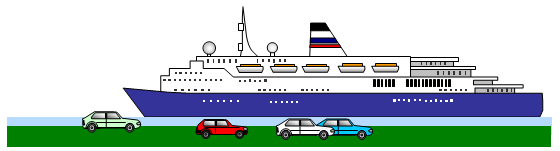You can use Archimedes principle to get a rough idea of the draught of a ship such as a ferry. In the following example we will consider the Bretagne of the Brittany Ferries fleet that sails across the English Channel between Britain and France.

Example problem
Mass = 24 500 metric tons = 24.5 x106 kg
Upthrust = weight of water displaced = 24.5x106g N
Density of sea water = 1030 kgm-3
Volume of water displaced = 24.5 x106/1030 = 2.38x104 m3
Length of ferry (assume a rectangular section) = 151 m
Width of ferry = 26 m Volume of ferry below the waterline = Area x draught = 151x26xdraught = 2.38x104
Therefore draught = 2.38x104/[151x26] = 6.06 m

(The actual draught of the ferry is 6.2 m, slightly different since we have assumed a rectangular cross section)

### Raising a block from a pond

A rectangular block of stone is raised at a constant speed from the bottom of a pond by a crane. Draw the variation in the tension of the cable from the moment the stone leaves the bottom of the pond to when the stone is completely clear of the water.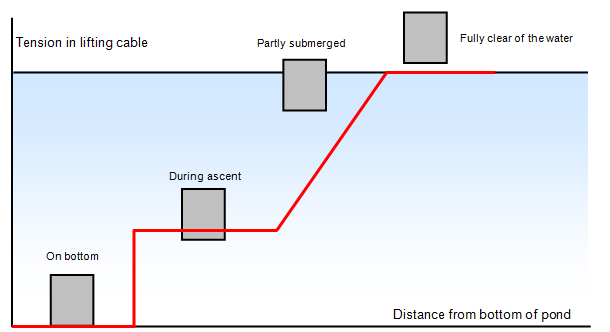### schoolphysics Archimedes animation

To see an animation of a cylinder being raised from a liquid click on the animation link.

A VERSION IN WORD IS AVAILABLE ON THE SCHOOLPHYSICS USB